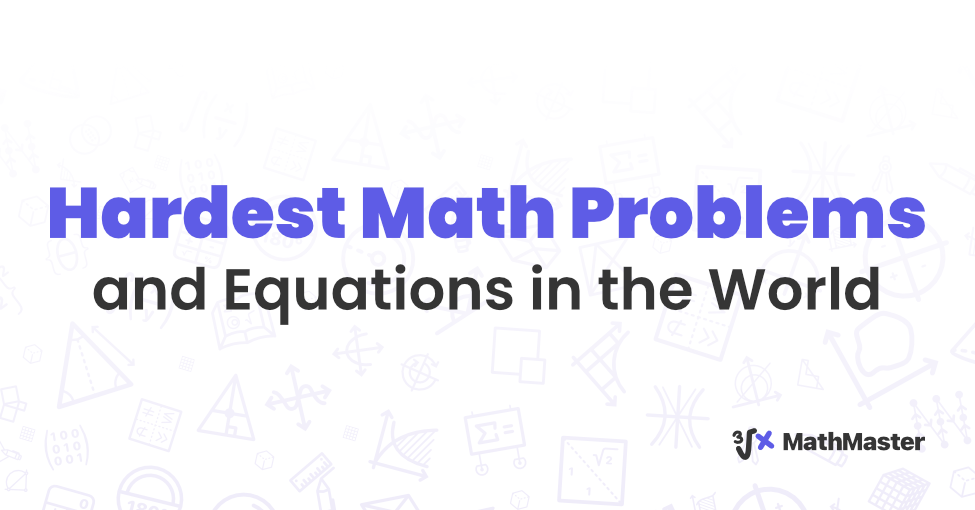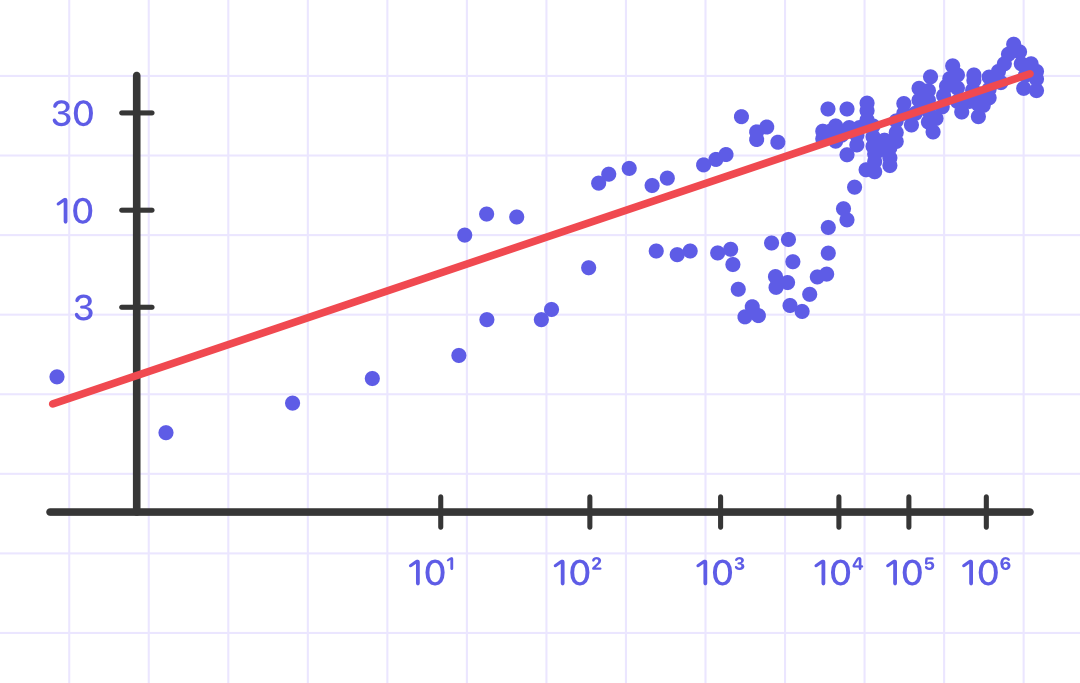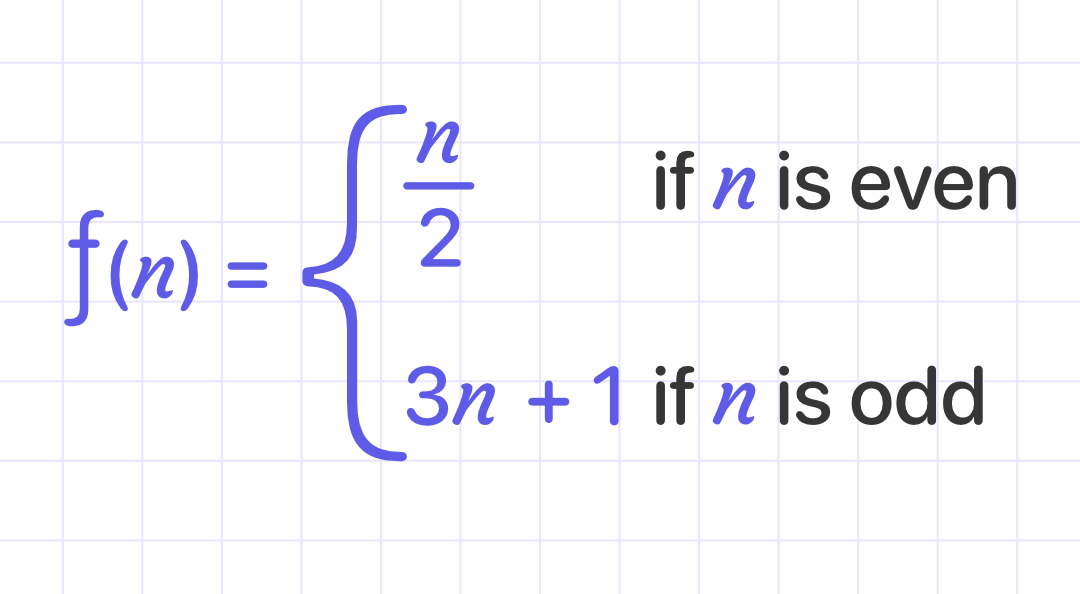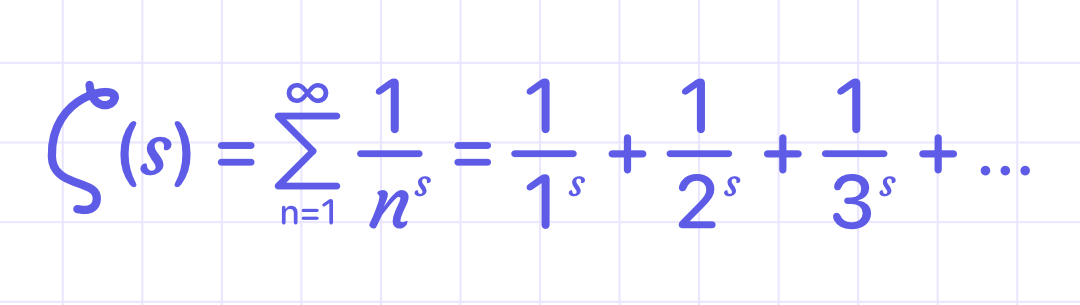# MathMaster BlogWhile countless math problems have been solved and helped improve ingenuity, a few are still considered the hardest math problem in the world. These equations have proven problematic to solve for many years and still trouble mathematicians to this day.

If you're wondering what is the hardest math problem in the world, then this is the right article for you.

## What Is The Hardest Math Problem In The World?

### The Reimann Hypothesis

The Riemann Hypothesis is arguably the most important open topic in all of the mathematics today, according to mathematicians. It is one of the seven Millennium Prize Problems, and whoever can solve it will receive a \$1 million award. Although it has significant ramifications for many areas of mathematics, it is also straightforward enough for us to cover the essentials right now.The function in the figure above is known as the Riemann zeta function.

For each s, this function gives an infinite sum, which takes some basic calculus to approach even the simplest values of s. For example, if s=2, then 𝜁$s$ is the well-known series 1 + 1/4 + 1/9 + 1/16 + …, which strangely adds up to exactly 𝜋²/6. When s is a complex number — one that looks like a+b𝑖, using the imaginary number 𝑖—finding 𝜁$s$ gets tricky.

It's so difficult, in fact, that it's dubbed the ultimate math problem. Specifically, the Riemann Hypothesis is about when 𝜁$s$=0; the official statement is, "Every nontrivial zeros of the Riemann zeta function has real part 1/2." On the plane of complex, this indicates that the function behaves in a specific way along a specific vertical axis. The idea is that the behavior keeps going in that direction indefinitely.

German mathematician Bernhard Riemann first introduced the Hypothesis and the zeta function in 1859. These were created by Riemann while researching the distribution of prime numbers. In the 160 years that have passed, our understanding of prime numbers has advanced significantly, and Riemann could never have predicted the power of today's supercomputers. The Riemann Hypothesis remains unsolved, which is a significant drawback.

A wave of new advancements would be possible if the Riemann Hypothesis were solved. It would generate a lot of buzz in the analysis and number theory fields. Until then, one of the biggest dams to the math research river continues to be the Riemann Hypothesis.

### The Collatz ConjectureThanks to the brilliant mathematician Terence Tao, word of work on this 82-year-old problem surfaced in September 2019. But unfortunately, even though Tao's narrative of success is encouraging, the issue hasn't yet been totally solved.

The Collatz Conjecture is all about that function f$n$, shown above, which takes even numbers and cuts them in half, while odd numbers get tripled and then added to 1. Take any natural number, apply f, then apply f again and again. You eventually land on 1 for every number we've ever checked. The Conjecture is that this is true for all natural numbers $positive integers from 1 through infinity$.

The Conjecture is a part of the field of mathematics known as Dynamical Systems, which examines systems that change over time in somewhat predictable ways. In several minor aspects, Tao's most recent work is a close approximation to the Collatz Conjecture. But as Tao went on to say, he probably can't change his approach to produce a comprehensive answer to the issue. Therefore, we may continue to work on it for many more years.

### The Birch and Swinnerton-Dyer ConjectureThe Birch and Swinnerton-Dyer Conjecture is one of the remaining six Millennium Prize Problems and one of the few that can be explained in simple terms. This conjecture relates to the mathematical subject of Elliptic Curves.

To put it briefly, an elliptic curve is a unique type of function. They take the unthreatening-looking form y²=x³+ax+b. It turns out that some characteristics of functions like this shed light on areas of mathematics like algebra and number theory.

In the 1960s, British mathematicians Peter Swinnerton-Dyer and Bryan Birch developed their conjecture. Its precise formulation is highly technical and has changed over time. Sir Andrew Wiles has been one of the principal guardians of this progress.

## The Bottom Line On The Hardest Math Problem In The World

Whether these math problems will be solved or not is still uncertain. Even if these equations are solved, there will always be another that becomes the hardest math problem in the world. For now, when you ask yourself what is the hardest math problem in the world, these are some of the questions you can consider.

For those equations that do have solutions but still feel like the hardest math problem, students use apps like MathMaster for the solution.